Copyright (C) 2012-present, The Computational Data Science Lab
https://github.com/cdslaborg/paramonte

# Making heatmap plots with the ParaMonte visualization tools

NOTE
If you are viewing an HTML version of this MATLAB live script on the web, you can download the corresponding MATLAB live script visualization_heatmap.mlx file to this HTML page at,
https://github.com/cdslaborg/paramontex/tree/main/MATLAB/mlx
Once you download the file, open it in MATLAB to view and interact with its contents, which is the same as what you see on this page.
First, let's clean up the MATLAB environment and make sure the path to the ParaMonte library is in MATLAB's path list.
clc;
clear all;
close all;
format compact; format long;
%%%%%%%%%%%% IMPORTANT %%%%%%%%%%%%%
% Set the path to the ParaMonte library:
% Change the following path to the ParaMonte library root directory,
% otherwise, make sure the path to the ParaMonte library is already added
% to MATLAB's path list.
pmlibRootDir = './';
%%%%%%%%%%%%%%%%%%%%%%%%%%%%%%%%%%%%
% change MATLAB's working directory to the folder containing this script
% if MATLAB Live Scripts did not create a temporary folder, we would not
% have all of these problems!
try
setwdFilePath = websave("setwd.m","https://github.com/cdslaborg/paramontex/raw/main/MATLAB/mlx/setwd.m");
run(setwdFilePath); % This is a MATLAB script that you can download from the same GitHub location given in the above.
catch % alas, we will have to run the simulations in MATLAB Live Script's temporary folder
filePath = mfilename('fullpath');
[currentDir,fileName,fileExt] = fileparts(filePath); cd(currentDir);
cd(fileparts(mfilename('fullpath'))); % Change working directory to source code directory.
end

## ParaMonte's default visualization tools

The ParaMonte library ships with several visualization tools that automate much of the MATLAB coding required to visualize the output of the simulations performed by the ParMonte library samplers.
By replacing the input dataFrame to these tools and following the conventions of the ParaMonte library, one can also use these visualization tools for any dataset that may not have been generated by the ParaMonte library.
Consider the following refined (decorrelated) sample from a MultiVariate Normal distribution, generated via the ParaDRAM sampler of the ParaMonte library by refining (decorrelating) its output Markov chain. Since this is a refined sample file as inidicated by its suffix "_sample.txt" We will read this file via the ParaDRAM sampler's readSample() method.
pm = paramonte();
This method automatically generates a set of tools that can be used to visualize the contents of the compact sample file. Note that these visualization tools are not unique to this particular method of the ParaDRAM sampler or other ParaMonte samplers. For the sake of illustration however, we will create heatmap plots using the above dataset read via readSample() method of the ParaDRAM routine.
When a sample or chain file is parsed via the readChain() or readSample() methods, an instance of HeatmapPlot() class is automatically added to the stats component of each element fo the output sampleList object from the parser. These instances are automatically preloaded with data for the corvariance and correlation matrices of the sample so that they can be readily plotted,
sample = pmpd.sampleList{1};
covmat = sample.stats.covmat
covmat =
CorCovMat with properties: columns: [2 3 4 5] plot: [1×1 struct] rows: [] df: [4×4 table]
cormat = sample.stats.cormat
cormat =
CorCovMat with properties: columns: [2 3 4 5] plot: [1×1 struct] rows: [] df: [4×4 table] method: "pearson"

## Plotting the corvariance matrix

covmat.plot.heatmap.make();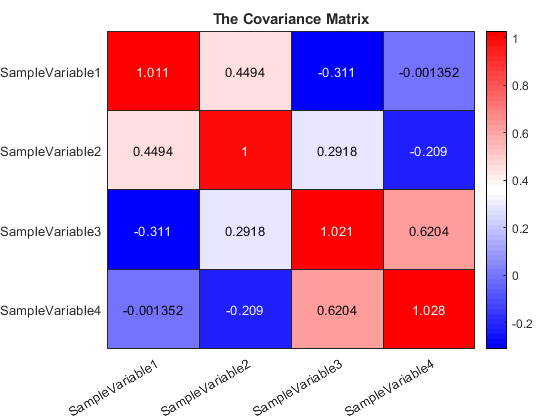Be default, the visualization tools are loaded with a set of predefined settings. These however, can be readily changed. For example, to change the colormap,
covmat.plot.heatmap.colormap.values
ans = 64×3
0 0 1.000000000000000 0.032258064516129 0.032258064516129 1.000000000000000 0.064516129032258 0.064516129032258 1.000000000000000 0.096774193548387 0.096774193548387 1.000000000000000 0.129032258064516 0.129032258064516 1.000000000000000 0.161290322580645 0.161290322580645 1.000000000000000 0.193548387096774 0.193548387096774 1.000000000000000 0.225806451612903 0.225806451612903 1.000000000000000 0.258064516129032 0.258064516129032 1.000000000000000 0.290322580645161 0.290322580645161 1.000000000000000
covmat.plot.heatmap.colormap.values = "winter";
covmat.plot.heatmap.make()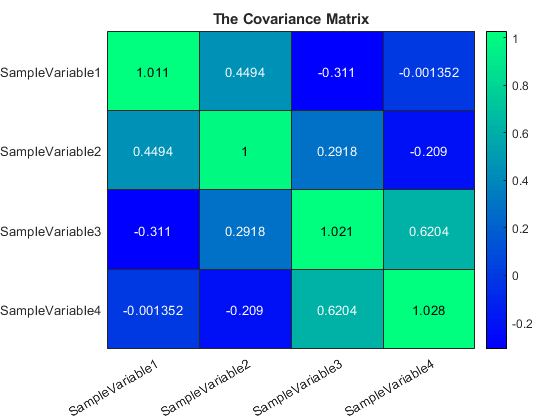covmat.plot.heatmap.reset(); % reset the properties of the plot object to the default settings.
ParaDRAM - NOTE: resetting the properties of the heatmap plot...
covmat.plot.heatmap.colormap.values = redblue();
covmat.plot.heatmap.heatmap.kws
ans = struct with fields:
colorbarVisible: "on" missingDataColor: [0.150000000000000 0.150000000000000 0.150000000000000] title: "The Covariance Matrix"
covmat.plot.heatmap.heatmap.kws.colorbarVisible = "off"; % turn the colorbar off
covmat.plot.heatmap.make();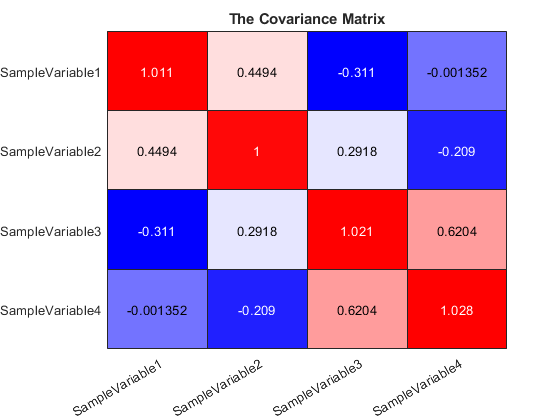Sometimes it makes sense to symmetrize the range of the limits of the colors used in the plot, for he sake of better visualization. This can be done via,
covmat.plot.heatmap.reset();
ParaDRAM - NOTE: resetting the properties of the heatmap plot...
covmat.plot.heatmap.make();
covmat.plot.heatmap.adjustColorLimits(); % symmetrizes the color limits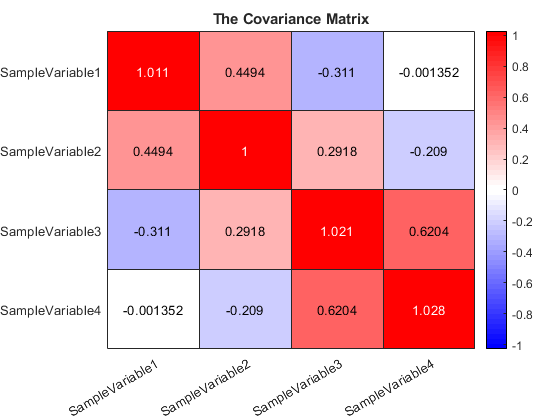Notice the change in colors and the range of the colorbar in this plot to symmetric range and compare it with the first plot we made in the above. If any other range is preferred, it can be passed to the same method,
covmat.plot.heatmap.reset();
ParaDRAM - NOTE: resetting the properties of the heatmap plot...
covmat.plot.heatmap.make();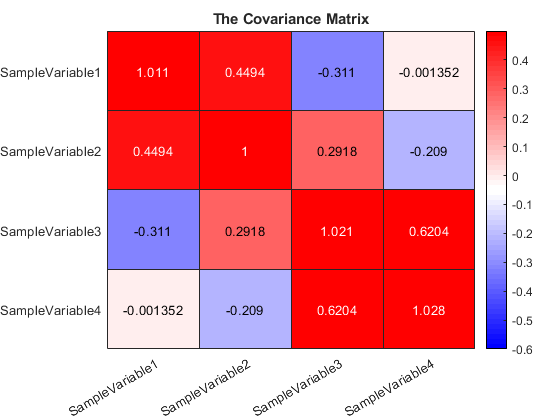## Controlling the precision of the values displayed

The precision of the heatmap matrix elements can be set via,
covmat.plot.heatmap.precision
ans = []
covmat.plot.heatmap.precision = 2;
covmat.plot.heatmap.make();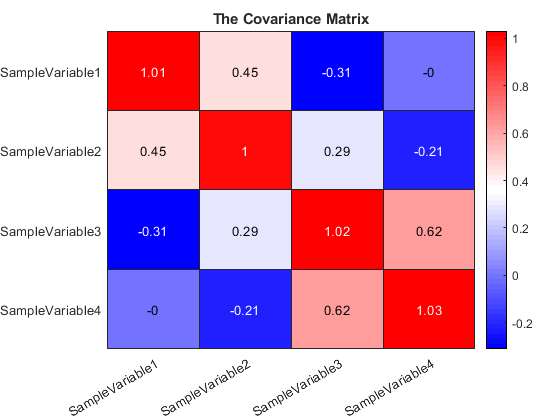## Reseting the plot to the default settings

To reset the properties of the line object to the default settings, try,
covmat.plot.heatmap.reset();
ParaDRAM - NOTE: resetting the properties of the heatmap plot...
To reset the entire line object including reading the data again from the input dataFrame, try,
covmat.plot.heatmap.reset("hard");
ParaDRAM - NOTE: creating the heatmap plot object from scratch...

## Plotting the correlation matrix

The syntax to plot the correlation matrix of the sample is almost identical to that of the correlation matrix,
cormat = sample.stats.cormat
cormat =
CorCovMat with properties: columns: [2 3 4 5] plot: [1×1 struct] rows: [] df: [4×4 table] method: "pearson"
cormat.plot.heatmap.make();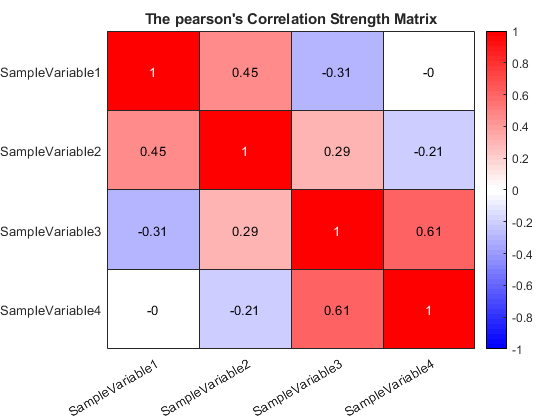Since this a correlation matrix, the range of the colorbar is automatically symmetrized to [-1 +1]. All settings and methods of the correlation matrix plot are identical to those of the covariance matrix heatmap plot discussed in the above.

## Exporting figures to external files

To extract a covariance or correlation heatmap plot to an external PNG file, call the exportFig() method of the corresponding object,
cormat.plot.heatmap.exportFig("exportedFigure.png","-m4")The above command will extract the current active figure to an output file with the relatively high resoluton as specified by the flag -m4. To make the exported figure smaller, one could specify -m2 instead. In addition, to generate a figures with background transparency, the flag -transparent can be added to the exportFig function call,
% covmat.plot.heatmap.exportFig("exportedFigure.png","-m2 -transparent") % uncomment to export the figure with transparency
Final Note:
To see other more sophisticated types of plots that can be automatically made with the ParaMonte visualization tools, visit: https://www.cdslab.org/paramonte/notes/examples/matlab/mlx/

{% comment %}
{% endcomment %}